# Assignment Help With The Material Balance Equation

### The Material Balance EquationThe material balance equation (MBE) has long been recognized as one of the basic tools of reservoir engineers for interpreting and predicting reservoir performance. The MBE, when properly applied, can be used to:

• Estimate initial hydrocarbon volumes in place
• Predict future reservoir performance
• Predict ultimate hydrocarbon recovery under various types of primary driving mechanisms

The equation is structured to simply keep inventory of all materials entering, leaving and accumulating in the reservoir. Simplest form, the equation can be written on volumetric basis as:

Initial volume = volume remaining + volume removed

Since oil, gas, and water are present in petroleum reservoirs, the material balance equation can be expressed for the total fluids or for any one of the fluids present. Before deriving the material balance, it is convenient to denote certain terms by symbols for brevity. The symbols used conform where possible to the standard nomenclature adopted by the Society of Petroleum Engineers.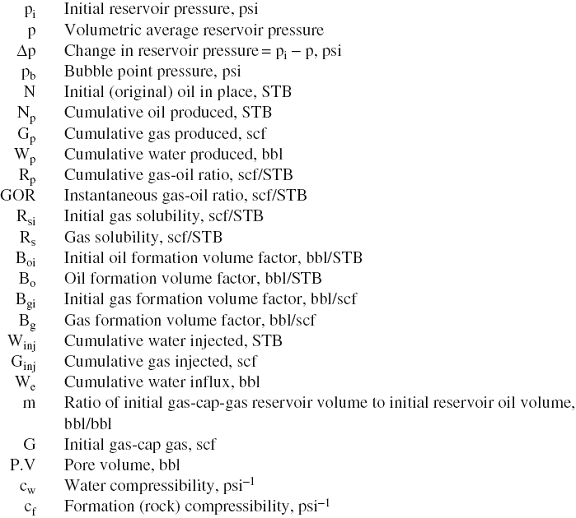Several of the material balance calculations require the total pore volume (P.V) as expressed in terms of the initial oil volume N and the volume of the gas cap. The expression for the total pore volume can be derived by conveniently introducing the parameter m into the relationship as follows:

Defining the ratio m as: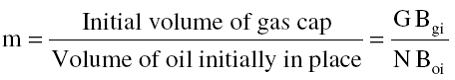Solving for the volume of the gas cap gives:

Initial volume of the gas cap = G Bgi = m N Boi

The total volume of the hydrocarbon system is then given by:

Initial oil volume + initial gas cap volume = (P.V) (1 - Swi)

N Boi + m N Boi = (P.V) (1 -Swi)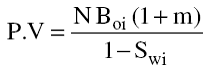Where

Swi = initial water saturation

N = initial oil in place, STB

P.V = total pore volume, bbl

m = ratio of initial gas-cap-gas reservoir volume to initial reservoir oil volume, bbl/bbl.

Volumetric balance expressions can be derived to account for all volumetric changes which occur during the natural productive life of the reservoir.

The MBE can be written in a generalized form as follows:

Pore volume occupied by the oil initially in place at pi +

Pore volume occupied by the gas in the gas cap at pi =

Pore volume occupied by the remaining oil at p +

Pore volume occupied by the gas in the gas cap at p +

Pore volume occupied by the evolved solution gas at p +

Pore volume occupied by the net water influx at p +

Change in pore volume due to connate water expansion and pore volume reduction due to rock expansion. +

Pore volume occupied by the injected gas at p +

Pore volume occupied by the injected water at p

The above nine terms composing the MBE can be separately determined from the hydrocarbon PVT and rock properties, as follows:

Pore Volume Occupied by the Oil Initially in Place.

Volume occupied by initial oil in place = N Boi

Where

N = oil initially in place, STB

Boi = oil formation volume factor at initial reservoir pressure pi, bbl/STB.

Pore Volume Occupied by the Gas in the Gas Cap

Volume of gas cap = m N Boi

Where m is a dimensionless parameter and defined as the ratio of gas-cap volume to the oil zone volume.

Pore Volume Occupied by the Remaining Oil

Volume of the remaining oil = (N - Np) Bo

Where

Np = cumulative oil production, STB

Bo = oil formation volume factor at reservoir pressure p, bbl/STB

Pore Volume Occupied by the Gas Cap at Reservoir Pressure p

As the reservoir pressure drops to a new level p, the gas in the gas cap expands and occupies a larger volume. Assuming no gas is produced from the gas cap during the pressure decline, the new volume of the gas cap can be determined as: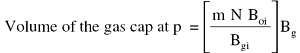Where

Bgi = gas formation volume factor at initial reservoir pressure, bbl/scf.

Bg = current gas formation volume factor, bbl/scf.

Pore Volume Occupied by the Evolved Solution Gas

This volumetric term can be determined by applying the following material balance on the solution gas: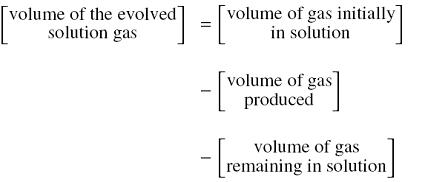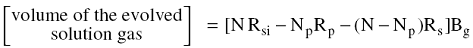Where

Np = cumulative oil produced, STB

Rp = net cumulative produced gas-oil ratio, scf/STB

Rs = current gas solubility factor, scf/STB

Bg = current gas formation volume factor, bbl/scf

Rsi = gas solubility at initial reservoir pressure, scf/STB

Pore Volume Occupied by the Net Water Influx

Net water influx =We -Wp Bw

Where

We = cumulative water influx, bbl

Wp = cumulative water produced, STB

Bw = water formation volume factor, bbl/STB